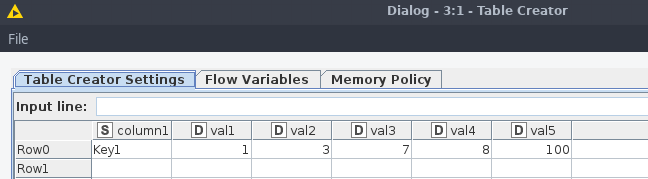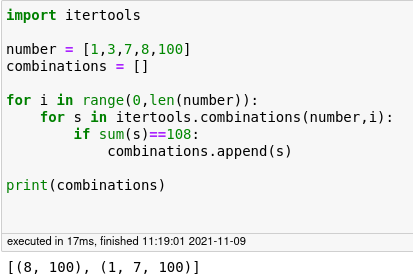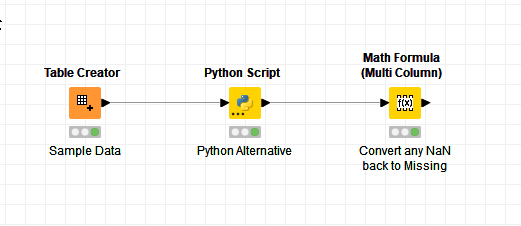# Find combination of columns summing to specific value

I have a table with a dynamic number of decimal columns. The goal is to find all combinations of columns which is summing to one specific value.

E.g.I want to list all columns which are summing to, say, 108.

Python snippet -I’ll appreciate any help or hint.

Thank you very much.

Hi @shubh,

To clarify what you are wanting to do, are we to assume that your data will actually have multiple rows, and so the combinations could be different on each row, but the target number will always be the same, or does the target number change for each row too?

When you say you have a table with a “dynamic number of columns” , what do you mean by that? Do you mean maybe that on any given row some columns are populated, and some are empty/missing? Can you provide an example of such a table?

Presumably the output would be in the form of an additional column containing a list, or a delimited string, to be appended on the end of each row?

Are you specifically looking for a python snippet to achieve the above or is the provided snippet just to give us an example of the logic?

2 Likes

Hi @takbb,

Thank you.

does the target number change for each row too? → each row has its own target number.

Sample data transformation -

recursive_loop_test.knwf (10.3 KB)

output - An observation column with a list of sets of column(s) that are summing to the target value.

Provided Python snippet was to give an example of the logic and I am trying to achieve the logic in Knime way.

Thanks again.

1 Like

Thanks for that clarification. It’s an interesting challenge that I’ll need to think about.

I haven’t got access to knime for much of today so I’m hopeful that somebody else will jump in here… I won’t mention names but I can think of a couple of people who might…In the meantime I’ll think this over as I haven’t got a solution in mind as yet. Be interesting to see what others come up with.

Hi @shubh

Challenging question. It reminds me of a forum post some time ago (see this wf on the hub )

For now I created this wf combinations.knwf (111.1 KB). The complexity that I didn’t solve, is how to handle the missing values in your columns, because now they are treated as if the value is 0. And that has an effect on the possible cat combinations. Please let us know if that is a (big) issue. The wf itself creates all possible combinations of column names. These combinations are used as a filter to calculate the sum of these combinations, and check if it equal to the target value.

gr. Hans

3 Likes

why not using the python snippet? This would probably require loops to check each combination and this is hardly faster or shorter to implement then just using your current solution.
br

2 Likes

I added some logic to the worklfow. Now it handles the columns with missing values (assuming the missing values are located at the end of the record). See: combinations_2.knwf (157.8 KB).

I needed a lot of nodes indeed and I’m curious about smarter, simpler solutions!
gr. Hans

1 Like

Hi @shubh,

I’m inclined to agree that this problem is probably best solved using a python node, based on the logic that you supplied. However, a challenge is a challenge…So, having decided that I couldn’t find any “quick wins” using nodes designed specifically for this type of task, I considered how to firstly derive the combinations. That is actually relatively easy, since you have a set of data columns and each is to either be included or excluded from each iteration, so its a binary decision. The total combinations from n columns is therefore “(2 raised to the power of n) minus 1”. Minus 1 because we exclude where no columns are involved. We assume we always want to include at least one column.

So there is at least one loop required here (the number of combinations), and as you increase the number of columns, you keep doubling the combinations and so this process will quickly become horribly slow, but assuming you have powerful tech, or plenty of time, here goes…The first few nodes prior to the loop simply count the column names of the form “catn_Value” and determines the number of iterations required to achieve the combinations. In the case of the sample table, there are 5 data columns, and therefore there will be `2^5 - 1 = 31` iterations.

For each iteration, an output column name is derived, which will be OutTotaln where n is between 1 and in this case 31.

The fun part in all this is the Column Expression node. This contains a further loop of its own, as it must loop through each of the columns.

For each combination (1 to 31), it must determine which columns (1 to 5) should be included. It uses the binary version of the iteration to do this, treating each column number (1 to 5) as if it were a “bit” .
The binary representations of 1 to 31 are:

``````1  = 00001
2  = 00010
3  = 00011
4  = 00100
...
...
28 = 11100
29 = 11101
30 = 11110
31 = 11111
``````

and this is useful as it tells us the combination of columns to include for each iteration. “1”=include, “0”=exclude.

The `Column Expressions` node is basically javascript, and (because I’m lazy!) I got a quick javascript snippet which determines whether the kth bit is set in a given number from here:

This node loops through each of the “value columns” and if, for that column number (1-5), the bit is set for the current combination-iteration (1-31), it adds the value of that column to a running total. If at the end, the total is the same as the target value column in the data table, it returns the values for that combination of columns. If it doesn’t it discards it.

``````n=variable("currentIteration")+1
s=""
tot=0
delim=""
for(colno=1; colno<=variable("Number Columns");colno++)
{

if (not(isMissing(column("cat"+colno+"_Value"))))
{
if ((n & (1 << (colno - 1))) > 0)
// if the bit represented by colno  is set in the current iteration n, then include
// the value of the colno column in the total
{
tot=tot+column("cat"+colno+"_Value");
s=s+delim+column("cat"+colno+"_Value");
delim="+"
}
}
}
if (tot==column("Target_Value"))
{s}
else
{""}
``````

This is then performed in the loop for each combination.

At the end, we have a series of columns representing the result for each iteration. This is then aggregated to keep just those where an actual (no empty) result was returned, and it is appended back onto the original data set.

Column Combinations.knwf (37.2 KB)

2 Likes

@shubh and here is an equivalent python script borrowing from your original post and making it work with the KNIME input table.``````import itertools

# Copy input to output
output_table_1 = input_table_1.copy()
flow_variables['knime.workspace']

numbers = output_table_1.filter(regex="cat.*_Value|Target_Value*", axis=1)

"""
Assumes that the "Target_Value" column will be the first column
in the "numbers" dataframe.

So it finds all combinations of cols from second column onwards
that sum to equal the first column

"""

df=numbers.apply(lambda row:
[s for i in range(len(row))
for s in itertools.combinations(row[1:], i)
if sum(s)==row ]
, axis=1)

"""
Creates a new column called "sets" to contain the sets created as a dataframe above
and sets its type as a string.
"""
output_table_1["sets"]=df
output_table_1.sets=output_table_1.sets.astype("string")

``````

This is (not surprisingly) significantly faster than my “standard nodes” offering.

Column Combinations - python.knwf (10.7 KB)

3 Likes

Hi @HansS, Thanks a lot. Sorry for the late reply, got occupied with festivals. Let me try this workflow.

2 Likes

Hi @takbb,Thanks a lot. Let me try to digest.

1 Like

This topic was automatically closed 182 days after the last reply. New replies are no longer allowed.